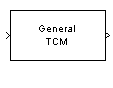# General TCM Encoder

Convolutionally encode binary data and map using arbitrary constellation

## Library

TCM, in Digital Baseband sublibrary of Modulation

•## Description

The General TCM Encoder block implements trellis-coded modulation (TCM) by convolutionally encoding the binary input signal and mapping the result to an arbitrary signal constellation. The Signal constellation parameter lists the signal constellation points in set-partitioned order. This parameter is a complex vector with a length, M, equal to the number of possible output symbols from the convolutional encoder. (That is, log2M is equal to n for a rate k/n convolutional code.)

### Input Signals and Output Signals

If the convolutional encoder represents a rate k/n code, then the General TCM Encoder block's input must be a binary column vector with a length of L*k for some positive integer L.

This block accepts a binary-valued input signal. The output signal is a complex column vector of length L. For information about the data types each block port supports, see Supported Data Types.

### Specifying the Encoder

To define the convolutional encoder, use the Trellis structure parameter. This parameter is a MATLAB® structure whose format is described in Trellis Description of a Convolutional Code. You can use this parameter field in two ways:

• If you want to specify the encoder using its constraint length, generator polynomials, and possibly feedback connection polynomials, then use a `poly2trellis` command within the Trellis structure field. For example, to use an encoder with a constraint length of 7, code generator polynomials of 171 and 133 (in octal numbers), and a feedback connection of 171 (in octal), set the Trellis structure parameter to

`poly2trellis(7,[171 133],171)`

• If you have a variable in the MATLAB workspace that contains the trellis structure, then enter its name as the Trellis structure parameter. This way is faster because it causes Simulink® software to spend less time updating the diagram at the beginning of each simulation, compared to the usage in the previous bulleted item.

The encoder registers begin in the all-zeros state. You can configure the encoder so that it resets its registers to the all-zeros state during the course of the simulation. To do this, set the `Operation mode` to Reset on nonzero input via port. The block then opens a second input port, labeled `Rst`. The signal at the `Rst` port is a scalar signal. When it is nonzero, the encoder resets before processing the data at the first input port.

### Signal Constellations

The trellis-coded modulation technique partitions the constellation into subsets called cosets so as to maximize the minimum distance between pairs of points in each coset.

Note

When you set the Signal constellation parameter, you must ensure that the constellation vector is already in set-partitioned order. Otherwise, the block might produce unexpected or suboptimal results.

As an example, the diagram below shows one way to devise a set-partitioned order for the points for an 8-PSK signal constellation. The figure at the top of the tree is the entire 8-PSK signal constellation, while the eight figures at the bottom of the tree contain one constellation point each. Each level of the tree corresponds to a different bit in a binary sequence (b3,b2,b1), while each branch in a given level of the tree corresponds to a particular value for that bit. Listing the constellation points using the sequence at the bottom of the tree leads to the vector

`exp(2*pi*j*[0 4 2 6 1 5 3 7]/8)`

which is a valid value for the Signal constellation parameter in this block.For other examples of signal constellations in set-partitioned order, see  or the reference pages for the M-PSK TCM Encoder and Rectangular QAM TCM Encoder blocks.

### Coding Gains

Coding gains of 3 to 6 decibels, relative to the uncoded case can be achieved in the presence of AWGN with multiphase trellis codes .

## Parameters

Trellis structure

MATLAB structure that contains the trellis description of the convolutional encoder.

Operation mode

In `Continuous` mode (default setting), the block retains the encoder states at the end of each frame, for use with the next frame.

In `Truncated (reset every frame)` mode, the block treats each frame independently. I.e., the encoder states are reset to all-zeros state at the start of each frame.

In `Terminate trellis by appending bits` mode, the block treats each frame independently. For each input frame, extra bits are used to set the encoder states to all-zeros state at the end of the frame. The output length is given by $y=n\cdot \left(x+s\right)/k$, where x is the number of input bits, and (or, in the case of multiple constraint lengths, s =`sum(ConstraintLength(i)-1)`). The block supports this mode for column vector input signals.

In `Reset on nonzero input via port` mode, the block has an additional input port, labeled `Rst`. When the `Rst` input is nonzero, the encoder resets to the all-zeros state.

Signal constellation

A complex vector that lists the points in the signal constellation in set-partitioned order.

Output data type

The output type of the block can be specified as a `single` or `double`. By default, the block sets this to `double`.

## Supported Data Types

PortSupported Data Types

Input

• Double-precision floating point

• Single-precision floating point

• Boolean

• 8-, 16-, and 32-bit signed integers

• 8-, 16-, and 32-bit unsigned integers

• ufix(1)

Output

• Double-precision floating point

• Single-precision floating point

## Pair Block

General TCM Decoder

## References

 Biglieri, E., D. Divsalar, P. J. McLane, and M. K. Simon, Introduction to Trellis-Coded Modulation with Applications, New York, Macmillan, 1991.

 Proakis, John G., Digital Communications, Fourth edition, New York, McGraw-Hill, 2001.

 Ungerboeck, G., “Channel Coding with Multilevel/Phase Signals”, IEEE Trans. on Information Theory, Vol IT28, Jan. 1982, pp. 55–67.

## Version History

Introduced before R2006a